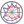Course title
Computer Simulation
 pham thi huongCourse description
This course introduces the fundamental and practical concepts of computer simulation as well as how to use MATLAB tool for handling and analyzing the simulation data. The topics include MATLAB programming, queue theory, etc. Assignments require an understanding of network problems and MATLAB programming.
Purpose of class
Students get familiar with MATLAB tool and obtain the ability to simulate and analyze the simulation result by using typical simulation technique.
Goals and objectives
1. Understanding the fundamental concepts of computer simulation
2. Understanding how to conduct a practical simulation to solve an engineering problem using MATLAB
3. Understanding how to handle and analyze the data
Language
English
Class schedule

Class schedule HW assignments (Including preparation and review of the class.) Amount of Time Required
1. Introduction to the Computer Simulation Consider what is the computer simulation, why is necessary to use, and where to use 100minutes
2. Introduction to the MATLAB Getting started with MATLAB environment 100minutes
3. Flow controls in MATLAB Study how to use flow controls in MATLAB 100minutes
4. User-defined functions Study how to build a large program in MATLAB and how to control random number generation 100minutes
5. Graphics How to analyze the output data 100minutes
6. File operation Study how to work with file input and output 100minutes
7. Queue theory and application of MATLAB in queue theory Study the fundamentals concept of queue theory 100minutes
8. First assignment and presentation Simulate a typical queue system 100minutes
9. Application of MATLAB in solving a network problem Introduce a network problem and build up the solution 100minutes
10. Second assignment and presentation Present the solution and discussion 100minutes
11. Introduce a specify Toolbox in MATLAB Introduce a useful toolbox in MATLAB 100minutes
12. Extension Build up the program according to the lecturer's instruction 100minutes
13. Summary of the course Summary of the course and discussion for the last presentation 100minutes
14. Final assignment and presentation Building the program and presentation 100minutes
Total. - - 1400minutes
Relationship between 'Goals and Objectives' and 'Course Outcomes'

Report Presentation Total.
1. 10% 15% 25%
2. 10% 15% 25%
3. 20% 30% 50%
Total. 40% 60% -
Evaluation method and criteria
Grades are based on students' simulation programs and presentation.
(An additional writing test can be used)
Textbooks and reference materials
1. A handout will be prepared
2. Official MATLAB website
Prerequisites
Some basic knowledge of programming language and network engineering.
Beginners are also welcome.
Office hours and How to contact professors for questions
• Appointment by email
• Tuesday, 10:50~12:30
Relation to the environment
Non-environment-related course
Regionally-oriented
Non-regionally-oriented course
Development of social and professional independence
• Course that cultivates an ability for utilizing knowledge
Active-learning course
Most classes are interactive
Course by professor with work experience
Work experience Work experience and relevance to the course content if applicatable
N/A N/A